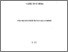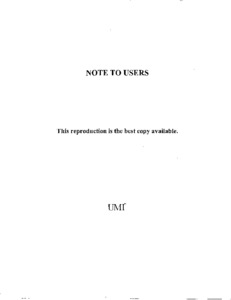Title:

An anytime deduction heuristic for first order probabilistic logic

Shahriar, Md. Istiaque (2008) An anytime deduction heuristic for first order probabilistic logic. Masters thesis, Concordia University.Preview
Text (application/pdf)
MR63162.pdf - Accepted Version
1MB

Abstract

This thesis describes an anytime deduction heuristic to address the decision and optimization form of the First Order Probabilistic Logic problem which was revived by Nilsson in 1986. Reasoning under uncertainty is always an important issue for AI applications, e.g., expert systems, automated theorem-provers, etc. Among the proposed models and methods for dealing with uncertainty, some as, e.g., Nilsson's ones, are based on logic and probability. Nilsson revisited the early works of Boole (1854) and Hailperin (1976) and reformulated them in an AI framework. The decision form of the probabilistic logic problem, also known as PSAT, consists of finding, given a set of logical sentences together with their probability value to be true, whether the set of sentences and their probability value is consistent. In the optimization form, assuming that a system of probabilistic formulas is already consistent, the problem is: Given an additional sentence, find the tightest possible probability bounds such that the overall system remains consistent with that additional sentence. Solution schemes, both heuristic and exact, have been proposed within the propositional framework. Even though first order logic is more expressive than the propositional one, more works have been published in the propositional framework. The main objective of this thesis is to propose a solution scheme based on a heuristic approach, i.e., an anytime deduction technique, for the decision and optimization form of first order probabilistic logic problem. Jaumard et al.  proposed an anytime deduction algorithm for the propositional probabilistic logic which we extended to the first order context.

Divisions: Concordia University > Gina Cody School of Engineering and Computer Science > Computer Science and Software Engineering Thesis (Masters) Shahriar, Md. Istiaque xii, 124 leaves : ill. ; 29 cm. Concordia University M. Comp. Sc. Computer Science and Software Engineering 2008 Jaumard, B 976310 Concordia University Library 22 Jan 2013 16:23 18 Jan 2018 17:42 http://clues.concordia.ca/search/c?SEARC...
All items in Spectrum are protected by copyright, with all rights reserved. The use of items is governed by Spectrum's terms of access.

Repository Staff Only: item control page

Back to top